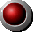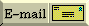The R-value of the Atmosphere
 Thermal Resistance R     the definition & the arcane units What's R for the atmosphere?       1/15 as much as for a house wall The rate at which heat passes through a wall is directly proportional to the surface area of the wall and also to the temperature difference between the two sides of the wall.  The formula is Heat rate = Area*(THi-TLo)/R, where R is the thermal resistance.  In the United States, it is (unfortunately) common to use the so-called British system of units, long since abandoned by the British.  In these units, temperature is measured in degrees Fahrenheit, the wall's area is measured in square feet, and the rate of heat passage is in British Thermal Units (BTU) per hour.  In these units, the R-value for the (nominally four-inch) fiberglass insulation used in normal house walls is 11.  The sheathing on the outside increases the R-value to about 12. So, what's the R-value of the earth's atmosphere?  The average temperature on the surface of the earth is around 15 degrees Celsius, and the temperature of the outer atmosphere is about -18 degrees C.  The difference, 33 degrees C is the same as 59 degrees Fahrenheit. We also know that 70% of the solar intensity (1350 watts per square meter) is absorbed by the earth.  The same amount of heat leaves the earth, passing (by many processes) through the atmosphere. We won't bore you with the arithmetic; indeed, we don't encourage anybody to use arcane units.  The result is that the R-value of the earth in the peculiar British units is about 0.8. The conclusion of this exercise:  The wall of an ordinary house has about 15 times as much thermal resistance as the earth's atmosphere.
To main Energy Advocate IndexYour comments & questions, please